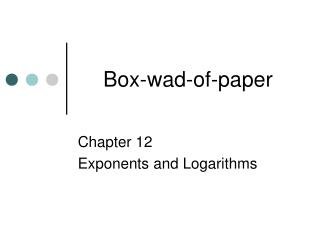DownloadDownload PresentationDownload Presentation- - - - - - - - - - - - - - - - - - - - - - - - - - - E N D - - - - - - - - - - - - - - - - - - - - - - - - - - -
##### Presentation Transcript

1. Box-wad-of-paper Chapter 12 Exponents and Logarithms

2. Box-wad-of-paper 29 1 5 9 13 17 21 25 30 2 6 10 14 18 22 26 3 7 11 15 19 23 27 4 8 12 16 20 24 28

3. Agenda • Get quiz back. • Work review problems from the board. (Turn them in at the end of class.) • Test tomorrow!!!!

4. 1 Solve. 52x = 59x + 7 x = -1

5. 2 Solve. x = -2, 5

6. 3 Solve. x = 1 6

7. 4 Solve. 27x = 800 x = ?

8. 5 Solve. logx 512 = 3 x = 8

9. 6 Solve. log8 (3x - 6) = log8 (9x + 23)

10. 7 Solve. log7 (x2 - 5) = log7 (59)

11. 8 Solve. log9(x + 3) = log92x x = ?

12. 9 Solve. Round to 4 decimal places. 3x = 72 x = 3.8928

13. 10 Solve. Round to 4 decimal places. 7x = 23.4 x = 1.6202

14. 11 Solve. Round to 4 decimal places. 53x = 37 x = 0.7479

15. 12 Write in logarithmic form. 82 = 64 log8 64 = 2

16. 13 Write in logarithmic form. 93 = 729 log9 729 = 3

17. 14 Write in logarithmic form.

18. 15 Write in logarithmic form. 65 = 7776 log6 7776 = 5

19. 16 Write in exponential form.

20. 17 Write in exponential form. log11 1331 = 3 113 = 1331

21. 18 Write in exponential form. log5 625 = 4 54 = 625

22. 19 Write in exponential form.

23. 20 Evaluate -4

24. 21 Evaluate 2

25. 22 Evaluate 4

26. 23 Expand log3 (27x)2 2(log3 27 + log3 x) = 2log327 + 2log3x

27. 24 Expand log x – log 9

28. 25 Condense

29. 26 Condense

30. 27 Evaluate. Round to 4 decimal places. log11 52 1.6478

31. 28 Evaluate. Round to 4 decimal places. log5 8.7 1.3441

32. 29 Evaluate. Round to 4 decimal places. log6 21.7 1.7175

33. 30 Evaluate. Round to 4 decimal places. log13 28.3 1.3033